1. Perimeter of circleCalculate the circumference of described circle to the triangle with sides 9,12,15 cm.
2. Percentage and rectangleAbout what percentage increases perimeter and area of a rectangle if both the sides 12 cm and 10 cm long we increase by 20%?
3. PercentCalculate how many % is the number 26.25 less than the number 105.
4. Ascent and descent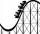Car goes 114 km track, which consists of ascent and descent at time of 1 hour 35 minutes. When climbing moves at the speed 48 km/h and downhill 25 m/s. What is the length of climb and descent?
5. Triangular prismBase of perpendicular triangular prism is a right triangle with leg length 5 cm. Content area of the largest side wall of its surface is 130 cm² and the height of the body is 10 cm. Calculate its volume.
6. Numbers at ratio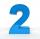The two numbers are in a ratio 3:2. If we each increase by 5 would be at a ratio of 4:3. What is the sum of original numbers?
7. Unknown number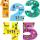Determine the unknown number, which double of its fourth square is equal the fifth its square.
8. Trapezium ABCDIn the figure, ABDC is a trapezium in which AB || CD. line segments RN and LM are drawn parallel to AB such that AJ=JK=KP. If AB=0.5m and AP=BQ=1.8m, find the lengths of AC, BD, RN and LM. angle D=angle C=60
9. Hexagon = 8 parts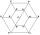Divide the regular hexagon into eight equal parts.
10. Square - increased perimeterHow many times is increased perimeter of the square, where its sides increases by 150%? If the perimeter of square will increase twice, how much% increases the content area of the square?
11. Volume of three cuboids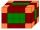Calculate the total volume of all cuboids for which the the size of the edges are in a ratio of 1:2:3, and one of the edges has a size 6 cm.
12. Three drivers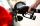Three driversdriving the same direction found that they have same amounth of gasoline. The first is enough to go 6 km, 4 km second and third 3km. Gasoline they divided so all three just drove to the nearest petrol station. How many km away was a petrol st
13. Desribed circle to rectangle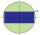Rectangle with sides 6 cm and 4 cm was circumscribed circle. What part of the content of the circle determined by the circumscribed circle occupies a rectangle? Express in perctentages(%).
14. Triangle KLBIt is given equilateral triangle ABC. From point L which is the midpoint of the side BC of the triangle it is drwn perpendicular to the side AB. Intersection of perpendicular and the side AB is point K. How many % of the area of the triangle ABC is area
15. RectangleThe length of the rectangle are in the ratio 5:12 and the circumference is 238 cm. Calculate the length of the diagonal and area of rectangle.
16. AP - simpleFind the first ten members of the sequence if a11 = 132, d = 3.
17. PrismFind the volume and surface area of prism with base of an equilateral triangle with side 7 dm long and the body height of 1.5 m.
18. Decagon prism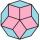A regular decagon of side a = 2 cm is the base of the perpendicular prism, the side walls are squares. Find the prism volume in cm3, round to two decimal places.
19. RingThe ring made from gold and copper alloy has a weight of 14.5 g and a volume of 1.03 cm3. How much gold and how much copper it contains? The metal densities are Au 19.3 g/cm³ and Cu 8.94 kg·dm-3In six baskets, the seller has fruit. In individual baskets, there are only apples or just pears with the following number of fruits: 5,6,12,14,23 and 29. "If I sell this basket," the salesman thinks, "then I will have just as many apples as a pear." Whic